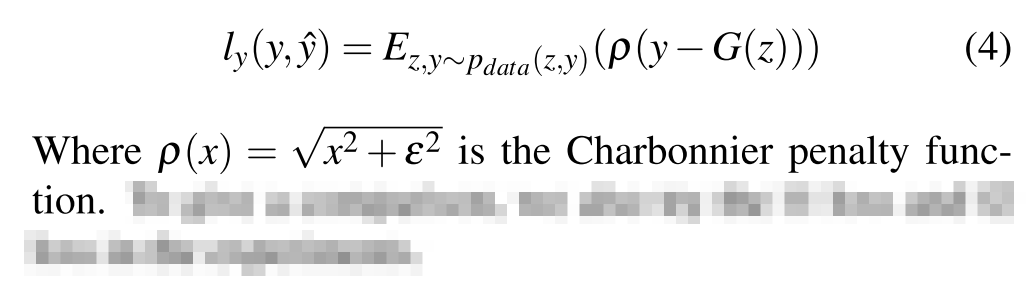# Making sense of charbonnier loss

I’m trying to make sense of a simple equation from this paper:What I don’t understand is p(x)=\sqrt{x^2 + \epsilon^2}. How am I supposed to find \epsilon (the error term I guess?) if p only takes x as input? Thanks

Just guessing not having read the paper, epsilon is probably a “small” hyperparameter to make sure the Charbonnier penalty is always non-zero (not sure why they want to ensure that, but that’s what it would do). You see that trick a lot to e.g. avoid dividing by zero.

1 Like

Thanks a lot, that make sense indeed

Here is another response from stackexchange.

I have no idea what this is about but I got curious.It’s unlikely that epsilon is used here just to make the penalty non-zero since you wouldn’t square it in that case.

The paper you linked to refers to “A More General Robust Loss Function” by Barron, which explains a bit more about this penalty function. Apparently it’s also known as the Pseudo-Huber loss, which has a Wikipedia page: https://en.wikipedia.org/wiki/Huber_loss

Anyway, the Barron paper refers to “Secrets of Optical Flow Estimation and Their Principles” by Sun et al. which refers to “Lucas/Kanade Meets Horn/Schunck: Combining Local and Global Optic Flow Methods” by Bruhn et al. which finally refers to “Two deterministic half-quadratic regularization algorithms for computed imaging” by Charbonnier and friends.

But I didn’t read all that because it had too much math. However, it seems that the Charbonnier loss is a mix between the L1 and L2 loss, depending on how you choose epsilon (usually called delta when talking about the Pseudo-Huber loss). If the error (x) is larger than epsilon, it acts like L1 regularization. If the error (x) is smaller than epsilon, it acts more like an L2 loss.

As your original paper says, they compared the Charbonnier penalty to both L1 and L2 regularization and found that it worked better. So epsilon is just another hyperparameter that you choose, just like how you’d choose your L1 or L2 (weight decay) hyperparameters. In the paper they chose epsilon=10^-8 (see section 4.1).

4 Likes

This is an L-1 norm penalty function p(x) = |x| which is not differentiable. So, epsilon is added as a small constant to make it differentiable.